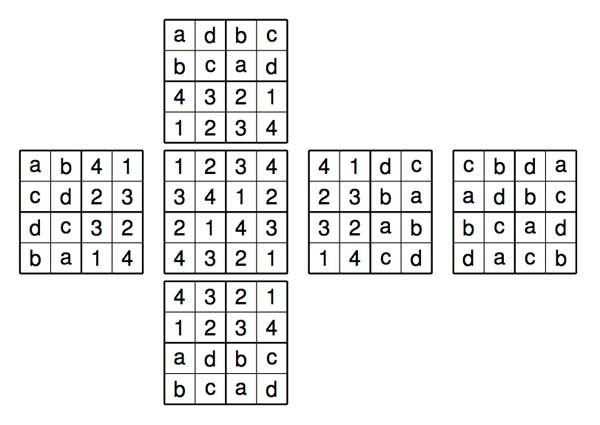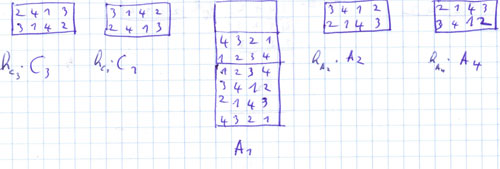Ibrahim Belkadi, one of my first-year group theory students invented the micro-sudokube, that is, a cube having a solution to a micro-sudoku on all its sides such that these solutions share one row along an edge. For example, here are all the solutions for a given central solution. There are 4 of them with ${ a,b } = { 2,3 }$ and ${ c,d } = { 1,4 }$The problem is : how many micro-sudokubes are there? Ibrahim handed in his paper and claims that there are exactly 32 of them, up to relabeling ${ 1,2,3,4 }$, so in all there are $32 \times 24 = 768$ micro-sudokubes.

The proof-strategy is as follows. Fix one side and use relabeling to put the solution on that side to be one of 12 canonical forms (see for example this post. Next, work out as above for each of these standard forms in how many ways it can be extended. A nice idea of Ibrahim was to develop a much better notation for micro-sudokubes than the above flattenet-out cube. He uses the fact that a micro-sudokube is entirely determined by the solutions on two opposite sides (check this for yourself). Moreover, fixing one side determines one-half of all the neighboring sides. His notation for the 4 solutions above then becomesand he can then use these solutions also in other standard form (the extra notation using the names A,B,C 1-4 for the 12 canonical forms).

Published in featured